# Nonlinear Magneto-Optical Rotation in an Alkali Atom

Here we examine the interaction of linearly polarized light and a longitudinal magnetic field with an atomic sample, as in the tutorial "Zeeman Structure: Nonlinear Magneto-Optical Rotation". In this case, we consider the D1 line of the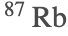atom, and write the input parameters in units appropriate for comparison with experimental results.

 Set options for DensityMatrix and plotting function.

First we set up the atomic system. We could enter the various atomic-state parameters by hand, but in this case it is convenient to pull them from a database. In this example we will focus on transitions originating from the ground-state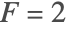hyperfine sublevel, and so we truncate the system by deleting the ground-statesublevels.

 Choose a transition. AtomicTransition just returns the names of the states associated with the given transition, which we can use to find the associated data in the AtomicData database.
 Get the list of quantum numbers and other information that we will use to set up the atomic system. We could also include the additional numerical atomic parameters in this list, but it can be more convenient to substitute their values in later.
 Create the list of sublevels in the atomic system. We specify BranchingRatio1, which means that the upperstate decays with 100% probability to the lowerstate. The individualbranching ratios are calculated automatically.
 DeleteStates removes the states satisfying the given criterion from the list of atomic states.
 Here are the remaining numerical parameters for the atomic system that we will substitute in later.

Now we form the Hamiltonian, relaxation and repopulation matrices, and Liouville equation assuming the steady-state condition.

 Form the Hamiltonian assuming an-polarized optical field with frequency ω and reduced Rabi frequency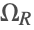, and a-directed magnetic field with nominal Larmor frequency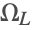.
 Draw the level diagram for the truncated system, showing the optical and magnetic-field interactions.
 Apply the rotating-wave approximation to the Hamiltonian, and write the optical frequency in terms of the detuning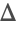.
 Create the matrix describing relaxation due to spontaneous decay and atomic transit.
 Create the matrix describing repopulation due to spontaneous decay and atomic transit.
 Form the Liouville equation for the steady-state density matrix.
 The list of density-matrix variables.

Now we find the changes in light polarization parameters in terms of the atomic density-matrix elements.

 The absorption, phase-shift, optical rotation, and change of ellipticity per unit path length and unit density.

Now we write the various input parameters in terms of experimentally relevant quantities.

 ExpandDipoleRME expresses the reduced dipole matrix element in terms of the natural width and energy of the transition.
 Convert ADM units to cgs by multiplying by, and then to SI by multiplying by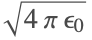.
 Write the optical electric field amplitude (in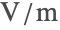) in terms of the light intensity (in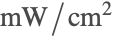).
 Write the Rabi frequency in terms of the light intensity.
 Write the Larmor frequency in terms of the magnetic field in Gauss.
 Write the total absorption and optical rotation in terms of the path length (in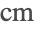), and the atomic density (in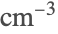).
 Substitute all of the above relations and the atomic parameters into the Liouville equation.

Finally, we plot the fractional electric-field amplitude absorption, and optical rotation (in radians). We can incorporate the effect of Doppler broadening by treating the Doppler shift as a shift in the light frequency. Thus the Doppler-broadened signal is given by the convolution of the Doppler-free signal and the Doppler profile.

 Plot the Doppler-free absorption and rotation signals at low magnetic field and fairly high light intensity to see the nonlinear magneto-optical effect. Note that for the chosen set of parameters there is significant power broadening of the Doppler-free signal.
 Convolve the signals with the Doppler distribution to obtain the Doppler-broadened signal.
 Choose higher magnetic field and lower light intensity to see the linear magneto-optical effect.
 Convolve the signal with the Doppler distribution.